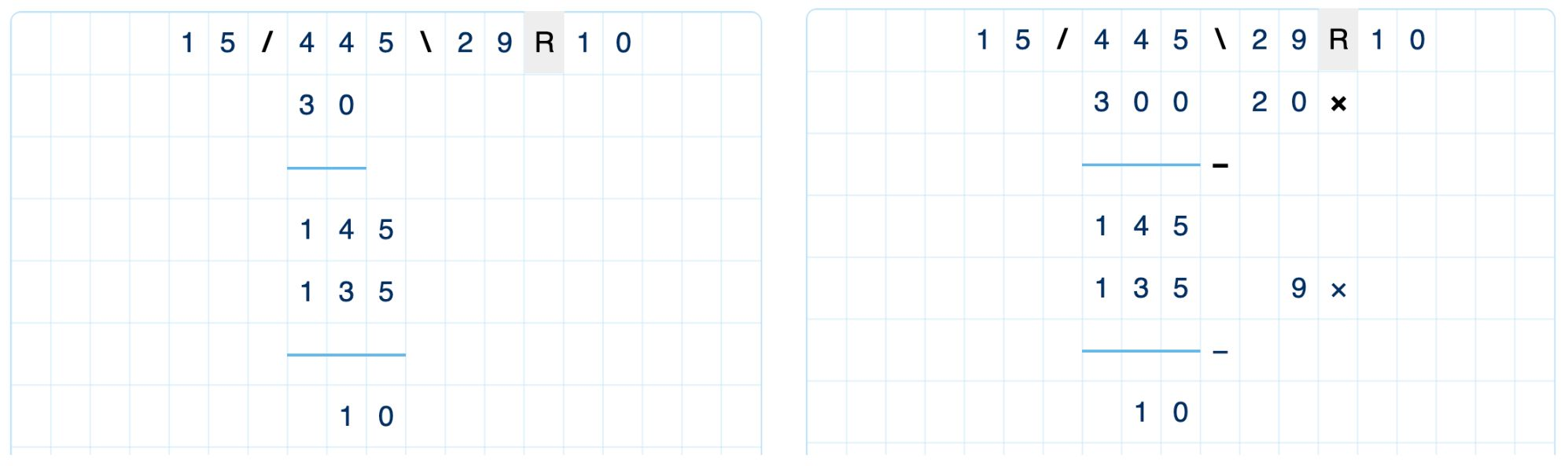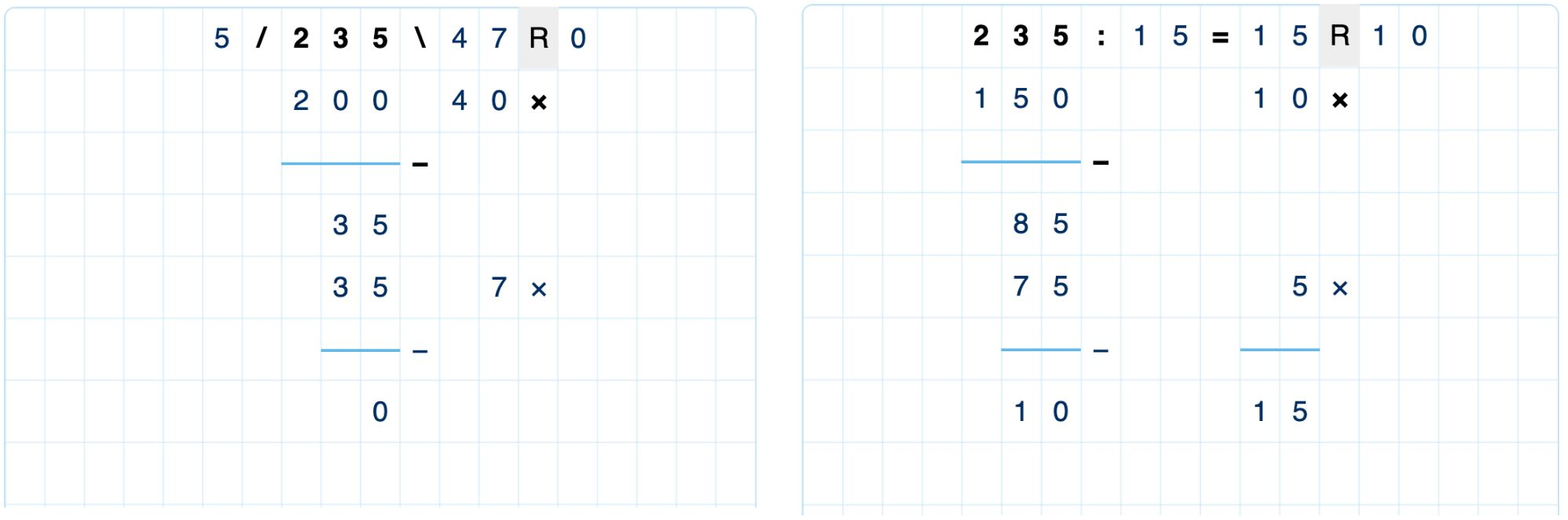# Multi-digit division

## Introduction

Algebrakit supports a short and extended form of multi-digit division, similar to the other calculation types. The short form of multi-digit division is called 'Long division.'

Note

Multi-digit multiplication does currently not support decimal numbers.Figure: Short and extended forms for division.

Students iteratively calculate the largest factor for each place value in the extended form. The answer is the sum of these intermediate factors.

The screenshot below shows two variations.

• You can choose from two notations of the division: using slashes (left) or division and equals signs (right).
• You can also configure whether students must find the sum of the factors through multi-digit addition (right).Figure: Two variations of the extended form of division.